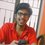# Distance covered by projectile

It so happened that since I secured full marks in a test of projectile motion, my teacher challenged me to find the answer to a question. Here it is:-

$$\color{blue}{\text{What is the distance covered by a projectile thrown with initial velocity}} \ \color{red}{u} \ \color{blue}{\text{and an angle of}} \ \color{red}{\theta} \ \color{blue}{\text{with the horizontal?}}$$

The first thing that came to my mind was the formula $\dfrac{u^2 \sin 2\theta}{g}$, but I realized that that was the formula for finding the horizontal range(displacement) by the projectile and not the distance.

Here is my approach for the problem:-

We break the trajectory into infinitely small bits such that it forms a right triangle of sides $dy$ and $dx$. Let the hypotenuse be $dl$.
Using Pythagoras' theorem:-
\begin{aligned} dl & = \sqrt{{(dx)}^2 + {(dy)}^2}\\ \\ dl & = \sqrt{{(dx)}^2\left(1 + {\left(\dfrac{dy}{dx}\right)}^2\right)}\\ \\ \text{Integrating on both sides}:-\\ \\ \int_{0}^{l} 1 \ dl & = \int_{0}^{R} \sqrt{\left(1 + {\left(\dfrac{dy}{dx}\right)}^2\right)} \ dx \end{aligned}

Note that $R$ here is the horizontal range of the projectile.

But my method seems too long. Is there any shorter method? Please post a shorter solution if there is one. (Air resistance is neglected).Note by Ashish Menon
5 years ago

This discussion board is a place to discuss our Daily Challenges and the math and science related to those challenges. Explanations are more than just a solution — they should explain the steps and thinking strategies that you used to obtain the solution. Comments should further the discussion of math and science.

When posting on Brilliant:

• Use the emojis to react to an explanation, whether you're congratulating a job well done , or just really confused .
• Ask specific questions about the challenge or the steps in somebody's explanation. Well-posed questions can add a lot to the discussion, but posting "I don't understand!" doesn't help anyone.
• Try to contribute something new to the discussion, whether it is an extension, generalization or other idea related to the challenge.

MarkdownAppears as
*italics* or _italics_ italics
**bold** or __bold__ bold
- bulleted- list
• bulleted
• list
1. numbered2. list
1. numbered
2. list
Note: you must add a full line of space before and after lists for them to show up correctly
paragraph 1paragraph 2

paragraph 1

paragraph 2

[example link](https://brilliant.org)example link
> This is a quote
This is a quote
    # I indented these lines
# 4 spaces, and now they show
# up as a code block.

print "hello world"
# I indented these lines
# 4 spaces, and now they show
# up as a code block.

print "hello world"
MathAppears as
Remember to wrap math in $$ ... $$ or $ ... $ to ensure proper formatting.
2 \times 3 $2 \times 3$
2^{34} $2^{34}$
a_{i-1} $a_{i-1}$
\frac{2}{3} $\frac{2}{3}$
\sqrt{2} $\sqrt{2}$
\sum_{i=1}^3 $\sum_{i=1}^3$
\sin \theta $\sin \theta$
\boxed{123} $\boxed{123}$

Sort by:

I think u might be looking for this...

- 5 years ago

Thanks!!!! For sharing, tht is of cool help.

- 5 years ago

i was supposed to find the same but was not asked by the teacher, i thought of it on my own and my answer is right but just one factor needed a little bit correction, thanks anyways........

Nice thought then! :P

- 5 years ago

I guess u are asked to find the range of the projectile since it is in horizontal distance. I suggest you make use of R=u^2sin2@/g

- 5 years ago

Nah, teacher explained the question first. Its not the horizontal range, its the length of the trajectory.

- 5 years ago

This is the only method.

However, here's a slight simplification: Substitute $x$ and $y$ in terms of the parameter $t$.

Hmm, ok i tried an alternative method too by using the formula of arc length of parabola, but that came out too tedious than this.

- 5 years ago

@Hung Woei Neoh @Swapnil Das @Rishabh Tiwari please do comment.

- 5 years ago

If I remember correctly the arc length of a curve is calculated this way

- 5 years ago

Yes it is, but i tried womething with eccentricity and something "stupid" :P it was a total mess.

- 5 years ago

i was supposed to find the same but was not asked by the teacher, i thought of it on my own and my answer is right but just one factor needed a little bit correction, thanks anyways........

- 4 years, 6 months ago

Thanks for the tip!

- 4 years, 6 months ago